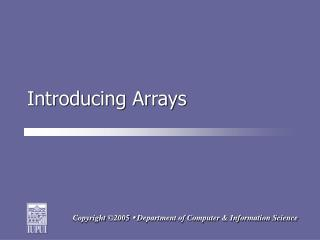Download PresentationIntroducing Arrays

# Introducing Arrays - PowerPoint PPT PresentationDownload Presentation## Introducing Arrays

- - - - - - - - - - - - - - - - - - - - - - - - - - - E N D - - - - - - - - - - - - - - - - - - - - - - - - - - -
##### Presentation Transcript

1. Introducing Arrays

2. Goals By the end of this lecture you should … • Understand when you would program an array. • Understand how to add values to array elements. • Understand how to use array subscripts. • Understand how to work with arrays in JavaScript.

3. Too Many Variables? • Remember, a variable is a data structure that can hold a single value at any given time. • What if I want to create an application to track student progress in a class with 50 students? Creating 50 variables will prove inefficient …

4. Introducing Arrays • An array might prove a better solution to my problem! • An array is a data structure that contains related items that are of the same data type and where each item in the array shares the same name. • In memory, array values occupy contiguous locations.

5. Multiple Variables in Memory strStu2 strStu3 strStu4 strStu5 strStu1 strStu7 strStu8 strStu6 strStu10 strStu11 strStu12 strStu9

6. An Array in Memory strWebPrgStudents

7. Array Elements & Subscripts • An element is a value stored in an array. JavaScript stores each element in a different position in an array. • We reference a position using a subscript (a.k.a. index). • To reference an element, we need to provide the array's name and the element's subscript:window.alert(strNames);

8. Index Order • We number subscripts starting with the number zero (0). We increment by 1 for each subsequent index. • The array’s length refers to the total number of indexes used in an array. The length is always one more than the last index number. Conversely, the last index number is always one less than the array’s length.

9. One-Dimensional Arrays • A one-dimensional array is a single list of elements subscripts. • Think of a seating chart an elementary school teacher might use. A single row of students is like a one-dimensional array.

10. 0 Janie Bobby 1 Sally 2 Joey 3 Mary 4 RowA Example of a 1D Array Mary is located in RowA

11. The Array Constructor • To create an array in JavaScript, we use the Array constructor method. • The Array constructor method provides us three distinct ways to create an array …

12. Creating an Empty Array • We can use the Array constructor to create an empty array, with no elements (we can add them later):var strStudents = new Array();

13. Dimensioning an Array • We can use the Array constructor to create an array of a specific size by supplying the Array constructor with an integer value representing an array's length:var strStudents = new Array(9);

14. Creating an Array with Specific Values • We can supply the Array constructor with specific values in a comma-delimited list:var strStudents = new Array("James","Ravi","Mary,"Jackson","Alyssa","Alexei");

15. Assigning Values to an Array • To assign a value to an array, you need to call the array by its name and then provide a subscript to store the new value (the index number goes in a pair of square brackets):strStudents = "Jakob";

16. Reading Array Values • To read a value from an array, call the array by name and indicate which subscript you want to retrieve (the index number goes in a pair of square brackets): window.alert(strStudents);

17. The array.length Attribute • We can find how many elements an array has by using the array.length attribute. array.length returns an integer value:intSum = strStudents.length;

18. Take the next few minutes to examine the file called introArrays_01.html.

19. Take the next few minutes to examine the file called introArrays_02.html.

20. Summary • Arrays are data structures that can hold multiple values at the same time. • The values in an array are called elements and they share the same name (the array's name). continued …

21. Summary • We indicate position in an array using a subscript. Subscript numbering begins with zero. • In JavaScript, we can create arrays using the Array constructor. • The Array.length attribute returns the size of an array.